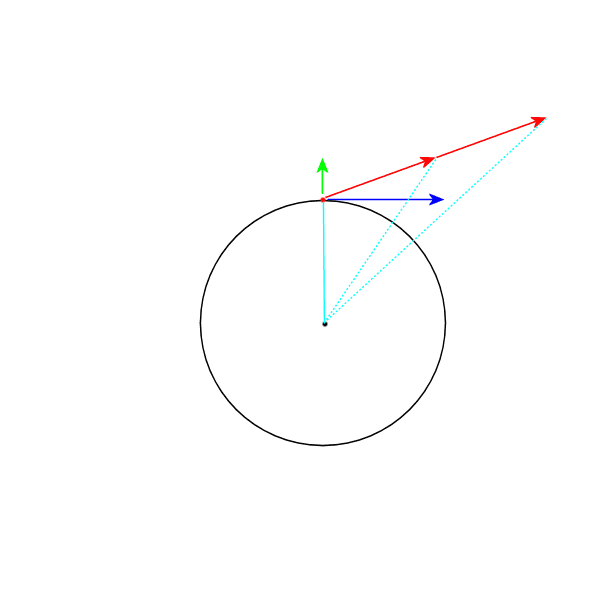# Jumping up on a rotating Earth

Guywithquestions
Hello,

I know it suppose to be a relatively basic question but still somehow I can't fully understand it.
Let assume that a man jumps vertically on the equator, while the Earth is of course rotating. What will happen to the value of his linear momentum in the horizontal axis?
It seems to me that if the angular momentum must be conserved, than the linear momentum must decrease, because:
J = p x r
And since r is increased due to the jump
p must decrease
Is it correct? If so how is it possible since both angular momentum and linear momentum must be conserved?

Thank you kindly.

Homework Helper
2022 Award
The angular and linear momentum of the system (earth+man) will be conserved (because there are by assumption ~no external forces or torques on the system). The man, depending upon how he jumps, can change momentum for himself (and exactly equally and oppositely for the earth).

•Delta2
Staff Emeritus
Gold Member
Summary: If a man jumps off the equator, What will happen to the value of his linear momentum in the horizontal axis?

Hello,

I know it suppose to be a relatively basic question but still somehow I can't fully understand it.
Let assume that a man jumps vertically on the equator, while the Earth is of course rotating. What will happen to the value of his linear momentum in the horizontal axis?
It seems to me that if the angular momentum must be conserved, than the linear momentum must decrease, because:
J = p x r
And since r is increased due to the jump
p must decrease
Is it correct? If so how is it possible since both angular momentum and linear momentum must be conserved?

Thank you kindly.
His linear momentum remains constant. So while the radius for calculating angular momentum increases his angular velocity with respect to the center of rotation must decrease.

Below is a quick vector diagram of the situation:
The green arrow is his Vertical jump speed
Blue arrow is his tangential velocity due to the rotation of the Earth
Red arrows are his resultant velocity broken into two sections of equal time.
Cyan lines represent the angles swept out by the radial line.
Note that for the first time section of his trajectory the cyan line has swept through a larger angle than it does during the second time section of equal length. The cyan line grows in length but rotates slower as time goes on.•lekh2003 and hutchphd
Guywithquestions